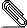# [Rdkit-discuss] handling of stereo information from mol files when not sanitizing

```Hi all

I would like to avoid sanitizing the sdf files, as information in these
files should be seen as the ground truth.```
```
I however have some problems in figuring out how to read and set chiral
information from the file and also have RDkit behave the same always.
Attached are two sdf files with no 3d information and only stereo
information in the atoms section for R-Aniline. The only difference as I
see it is the order of the lines of the bond information.
Even so I get two different smiles back with isomeric information when not
sanitizing.

Attached is also the minimal python code: which for me at least outputs:

not setting chiral flags
> CC(N)C(=O)O
> CC(N)C(=O)O
>
> setting chiral flags
> [H]OC(=O)[C@]([H])(N([H])[H])C([H])([H])[H]
> [H]OC(=O)[C@@]([H])(N([H])[H])C([H])([H])[H]
>
> setting chiral flags and sanitize
> C[C@@H](N)C(=O)O
> C[C@@H](N)C(=O)O
>

Any ideas to why this happens and how I can handle it strictly. Also what
does the sanitizing exactly do?

Regards Rasmus
```Ran2.sdf
Description: Binary dataRan1.sdf
Description: Binary data

```from rdkit import Chem

def set_correct_Chiral_flags(mol, debug=False):
for a in mol.GetAtoms():
if a.HasProp("molParity"):
try:
parity=int(a.GetProp("molParity"))
except ValueError:
parity=None
if parity and debug: print(a.GetSymbol(), a.GetIdx(), parity)
if parity==1:
a.SetChiralTag(Chem.rdchem.ChiralType.CHI_TETRAHEDRAL_CW)
elif parity==2:
a.SetChiralTag(Chem.rdchem.ChiralType.CHI_TETRAHEDRAL_CCW)
elif parity==3:
a.SetChiralTag(Chem.rdchem.ChiralType.CHI_UNSPECIFIED)
return mol

print('\nnot setting chiral flags and sanitizing')
print( Chem.MolToSmiles(Chem.MolFromMolFile('Ran1.sdf', removeHs=True, sanitize=True), isomericSmiles=True) )
print( Chem.MolToSmiles(Chem.MolFromMolFile('Ran2.sdf', removeHs=True, sanitize=True), isomericSmiles=True) )

print('\nsetting chiral flags')
print( Chem.MolToSmiles(set_correct_Chiral_flags(Chem.MolFromMolFile('Ran1.sdf', removeHs=True, sanitize=False)), isomericSmiles=True) )
print( Chem.MolToSmiles(set_correct_Chiral_flags(Chem.MolFromMolFile('Ran2.sdf', removeHs=True, sanitize=False)), isomericSmiles=True) )

print('\nsetting chiral flags and sanitize')
print( Chem.MolToSmiles(set_correct_Chiral_flags(Chem.MolFromMolFile('Ran1.sdf', removeHs=True, sanitize=True)), isomericSmiles=True) )
print( Chem.MolToSmiles(set_correct_Chiral_flags(Chem.MolFromMolFile('Ran2.sdf', removeHs=True, sanitize=True)), isomericSmiles=True) )

```
```_______________________________________________
Rdkit-discuss mailing list
Rdkit-discuss@lists.sourceforge.net
https://lists.sourceforge.net/lists/listinfo/rdkit-discuss
```